CBSE Class 12 Sample Paper for 2023 Boards

Class 12
Solutions of Sample Papers and Past Year Papers - for Class 12 Boards

## Find the mean number of defective items in a sample of two items drawn one-by-one without replacement from an urn containing 6 items, which include 2 defective items. Assume that the items are identical in shape and size.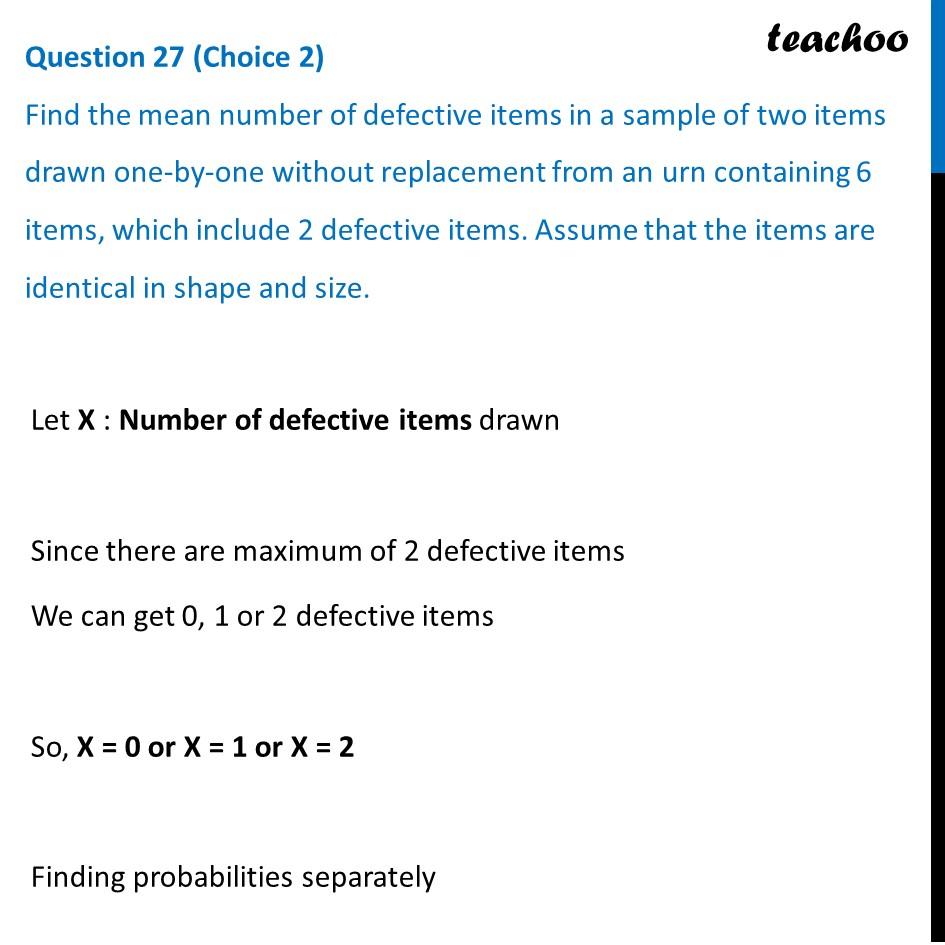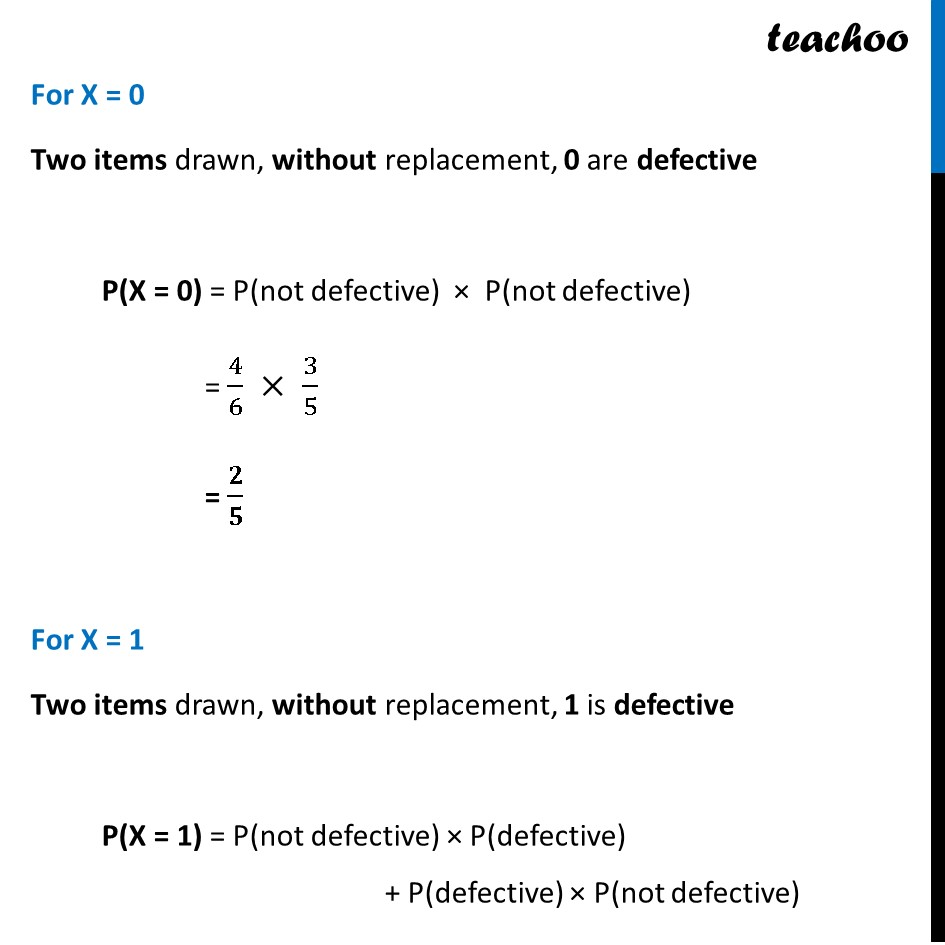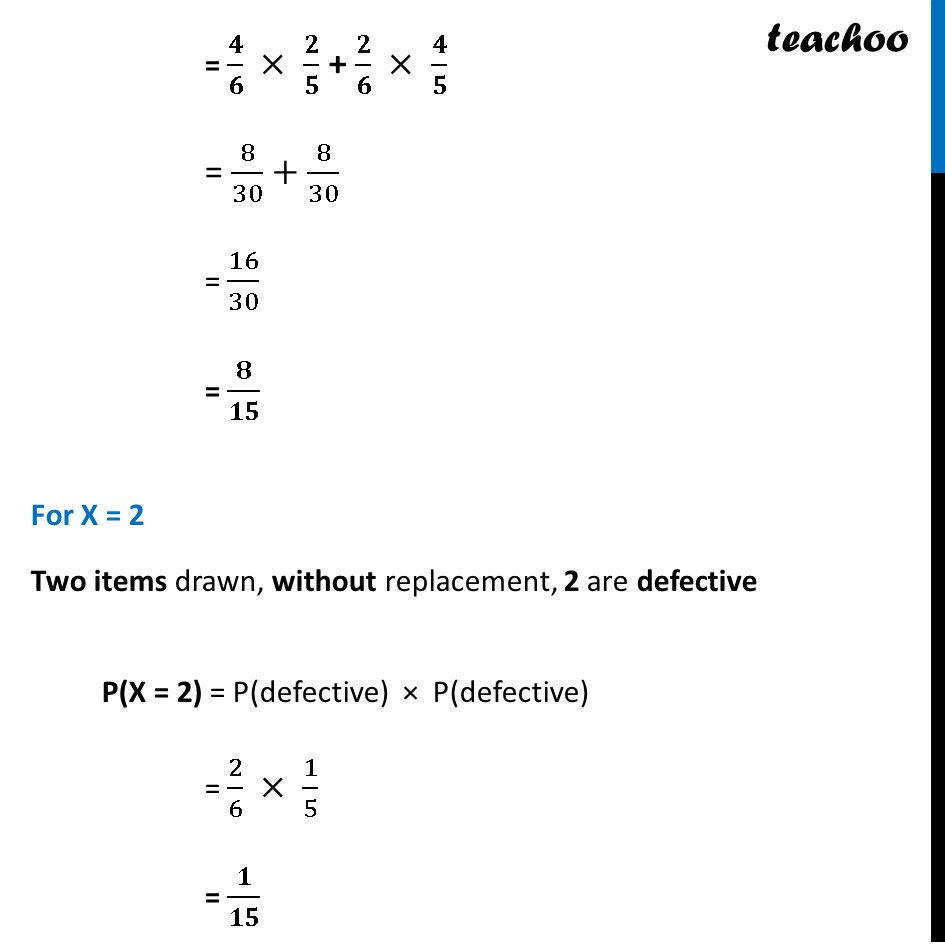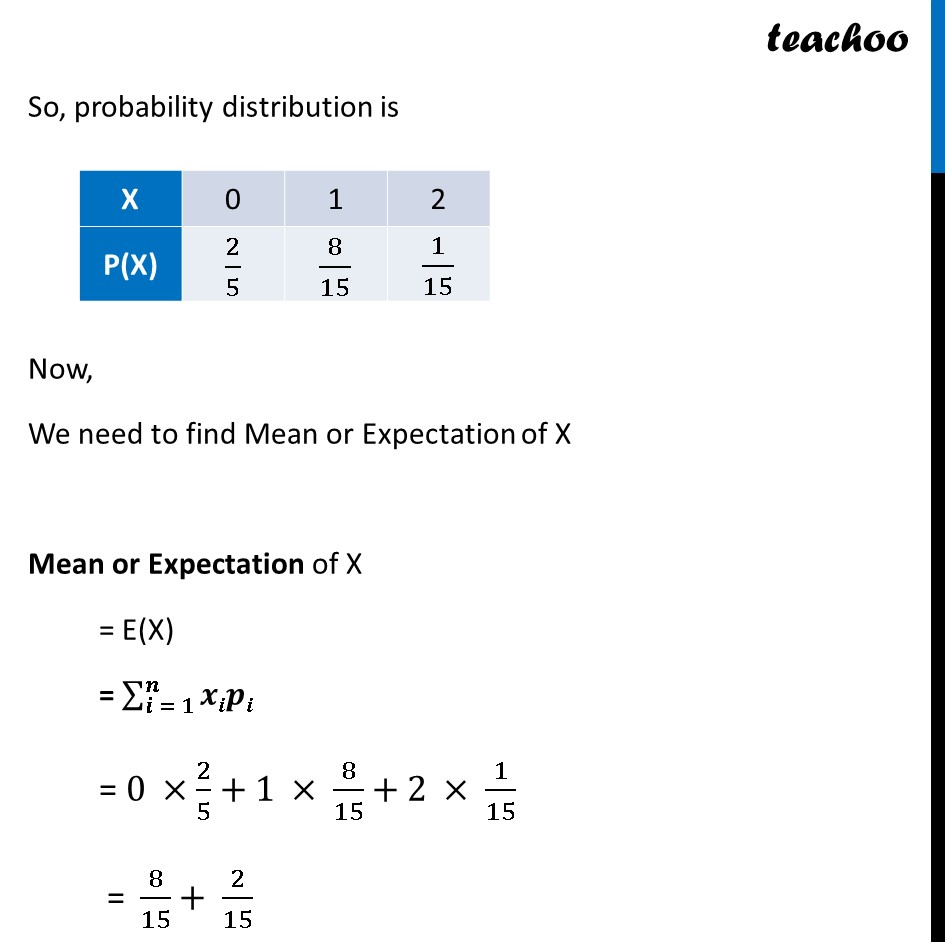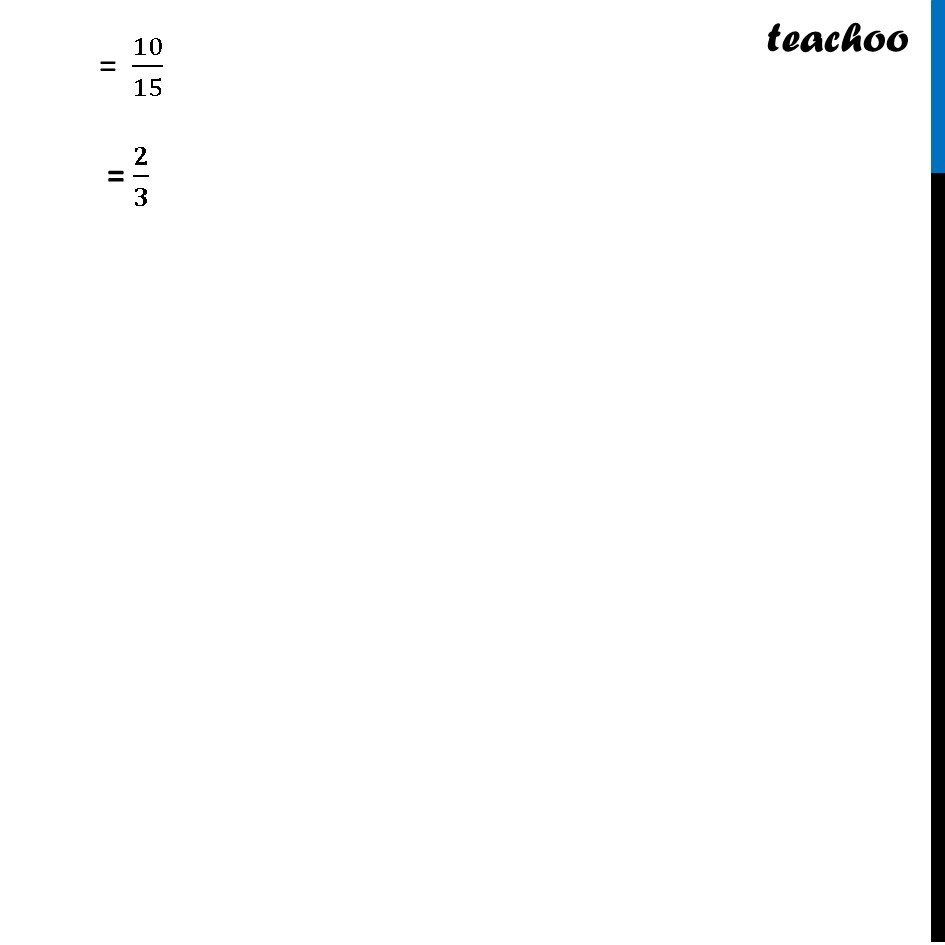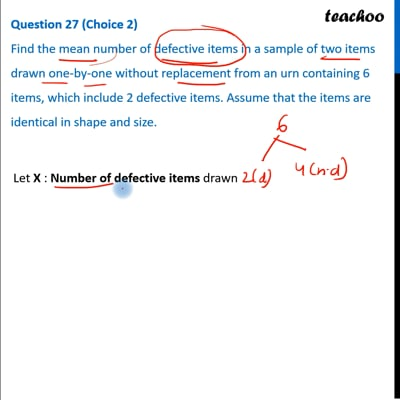This video is only available for Teachoo black users

Learn in your speed, with individual attention - Teachoo Maths 1-on-1 Class

### Transcript

Question 27 (Choice 2) Find the mean number of defective items in a sample of two items drawn one-by-one without replacement from an urn containing 6 items, which include 2 defective items. Assume that the items are identical in shape and size. Let X : Number of defective items drawn Since there are maximum of 2 defective items We can get 0, 1 or 2 defective items So, X = 0 or X = 1 or X = 2 Finding probabilities separately For X = 0 Two items drawn, without replacement, 0 are defective P(X = 0) = P(not defective) × P(not defective) = 4/6 × 3/5 = 𝟐/𝟓 For X = 1 Two items drawn, without replacement, 1 is defective P(X = 1) = P(not defective) × P(defective) + P(defective) × P(not defective) = 𝟒/𝟔 × 𝟐/𝟓 + 𝟐/𝟔 × 𝟒/𝟓 = 8/30+8/30 = 16/30 = 𝟖/𝟏𝟓 For X = 2 Two items drawn, without replacement, 2 are defective P(X = 2) = P(defective) × P(defective) = 2/6 × 1/5 = 𝟏/𝟏𝟓 So, probability distribution is Now, We need to find Mean or Expectation of X Mean or Expectation of X = E(X) = ∑_(𝒊 = 𝟏)^𝒏▒𝒙𝒊𝒑𝒊 = 0 ×2/5+1 × 8/15+2 × 1/15 = 8/15+ 2/15 = 10/15 = 𝟐/𝟑